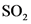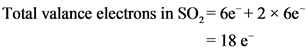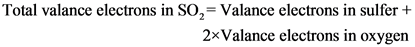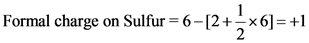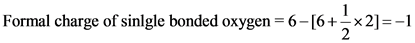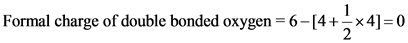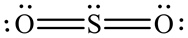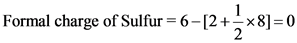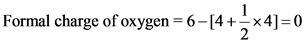# Draw a lewis structure for so2 in which all atoms obey the octet rule. show formal charges.

Draw a Lewis structure for SO2 in which all atoms obey the octet
rule. Show formal charges. Do not consider ringed structures. Draw a Lewis structure for SO2 in which all atoms have a formal
charge of zero. Explicitly showing the zero charges is optional. Do
not consider ringed structures.

General guidance

Concepts and reason
Calculate total number of valance electrons in and draw the Lewis structure that contains all the atoms with octet configuration.
Calculate formal charge by using the following formula.

Fundamentals

Lewis structure is a diagram that show bonding between atoms in a molecule and also shows lone pair of electrons present on the atoms.
Charge on an atom without considering electronegativity differences is called as formal charge.

Step-by-step

Step 1 of 3

Sulfur and oxygen atoms belong to Group 6A of the periodic table.

Sulfur belongs to group 6A of the periodic table. Thus, sulfur has 6 valance electrons atom is 6. Sulfur atom also has 6 valance electrons.

Total number of valance electrons is18.

Draw the Lewis structure of the molecule, verify octet of each atom of the molecule.

Step 2 of 3

Connect all the atoms of the molecule with respect of their valances. Correct Lewis structure with octet of all the atoms is as follows:

Lewis structure of with correct formal charges is as follows:

All the atoms in the molecule are connected with covalent bonds. Lone pairs of electrons are also added to each atom. Each atom of the molecule has octet.

Draw the Lewis structure for with formal charges of zero.

Step 3 of 3

Lewis structure with formal charges of zero is shown below.

Lewis structure with zero formal charges is
.

Calculate formal charges of the atoms.

Both the oxygen atoms have same number of unshared and shared electrons. So, they have formal charge of zero.
Thus, formal charges on all the atoms are zero.

Lewis structure of with correct formal charges is as follows:

Lewis structure with zero formal charges is
.

Lewis structure of with correct formal charges is as follows:

Lewis structure with zero formal charges is
.

Formal charge of atom= Number of valance electrons – [Number of unshared valance electrons + Number of shared valance electrons]
Total valance electrons in SO2 = 6e + 2 x 6e = 18 e
Total valance electrons in SO,= Valance electrons in sulfer + 2xValance electrons in oxygen
Formal charge on Sulfur = 6-[2+ = x6]=+1
Formal charge of sinlgle bonded oxygen = 6-1 -1
Formal charge of double bonded oxygen 6-[4+
ols.
Formal charge of Sulfur = 6–[2+-* *8]=0
Formal charge of oxygen =6-[4+x4]=0
Ö—
-0:
We were unable to transcribe this image